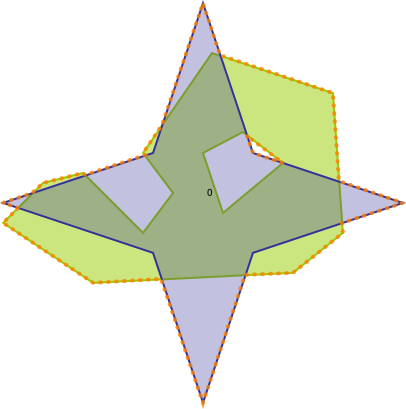#Boost C++ Libraries

...one of the most highly regarded and expertly designed C++ library projects in the world.

This is the documentation for an old version of Boost. Click here to view this page for the latest version.

#### union_

Combines two geometries which each other.

###### Description

The free function union calculates the spatial set theoretic union of two geometries.

###### Synopsis

```template<typename Geometry1, typename Geometry2, typename Collection>
void union_(Geometry1 const & geometry1, Geometry2 const & geometry2, Collection & output_collection)```

###### Parameters

Type

Concept

Name

Description

Geometry1 const &

Any type fulfilling a Geometry Concept

geometry1

A model of the specified concept

Geometry2 const &

Any type fulfilling a Geometry Concept

geometry2

A model of the specified concept

Collection &

output collection, either a multi-geometry, or a std::vector<Geometry> / std::deque<Geometry> etc

output_collection

the output collection

###### Header

Either

`#include <boost/geometry/geometry.hpp>`

Or

`#include <boost/geometry/algorithms/union.hpp>`

###### Conformance

The function union implements function Union from the OGC Simple Feature Specification.

NoteBoost.Geometry adds an underscore to avoid using the `union` keyword
###### Behavior

Case

Behavior

GeometryOut is a Polygon

Calculates union polygons of input (multi)polygons and/or boxes

NoteCheck the Polygon Concept for the rules that polygon input for this algorithm should fulfill
###### Example

Shows how to get a united geometry of two polygons

```#include <iostream>
#include <vector>

#include <boost/geometry.hpp>
#include <boost/geometry/geometries/point_xy.hpp>
#include <boost/geometry/geometries/polygon.hpp>
#include <boost/geometry/io/wkt/wkt.hpp>

#include <boost/foreach.hpp>

int main()
{
typedef boost::geometry::model::polygon<boost::geometry::model::d2::point_xy<double> > polygon;

polygon green, blue;

boost::geometry::read_wkt(
"POLYGON((2 1.3,2.4 1.7,2.8 1.8,3.4 1.2,3.7 1.6,3.4 2,4.1 3,5.3 2.6,5.4 1.2,4.9 0.8,2.9 0.7,2 1.3)"
"(4.0 2.0, 4.2 1.4, 4.8 1.9, 4.4 2.2, 4.0 2.0))", green);

boost::geometry::read_wkt(
"POLYGON((4.0 -0.5 , 3.5 1.0 , 2.0 1.5 , 3.5 2.0 , 4.0 3.5 , 4.5 2.0 , 6.0 1.5 , 4.5 1.0 , 4.0 -0.5))", blue);

std::vector<polygon> output;
boost::geometry::union_(green, blue, output);

int i = 0;
std::cout << "green || blue:" << std::endl;
BOOST_FOREACH(polygon const& p, output)
{
std::cout << i++ << ": " << boost::geometry::area(p) << std::endl;
}

return 0;
}
```

Output:

```green || blue:
0: 5.64795```
###### See also
 Copyright © 2009-2013 Barend Gehrels, Bruno Lalande, Mateusz Loskot, Adam Wulkiewicz Distributed under the Boost Software License, Version 1.0. (See accompanying file LICENSE_1_0.txt or copy at http://www.boost.org/LICENSE_1_0.txt)